# Biorobotics Laboratory BioRob

## A Kinematic Model for the iCub

Abstract

One of the research fields roboticists are working on nowadays is humanoid robotic. They try to develop humanoid robots in order to help old people alone at home or to understand some human behaviors like autism for example. Among all these different projects, there is the european RobotCub project which aims to study cognition through a robot looking like a two years old child and called iCub.
The goal of this semester project is to develop a kinematic model of the iCub that could later be used for studying biped stability during walking or for imposing kinematic constraints for example. First the iCub model under Webots has been updated according to the official model found on the wiki for the iCub (eris.liralab.it/wiki/ICubForwardKinematics). Secondly, the model is designed using an open source Kinematic and Dynamic Library called KDL (orocos.org/kdl). After this, the model is used to test the forward and inverse position kinematics algorithms provided by KDL. The results show that the forward position kinematics works well for kinematic chains and trees and that the inverse position for chains works well for chains (see videos "point6.avi", "circle2.avi" and "circle3.avi").

## Videos

iCub reaching a point without moving the torso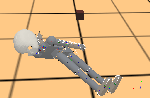iCub in a singular configuration (damping = lambda = 0; initial joint angles = 0) :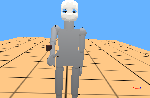effects of the damping :

damping = 0.1; initial joint angles = 0 :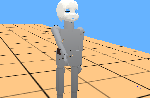damping = 0.2; initial joint angles = 0 :

damping = 0.3; initial joint angles = 0 :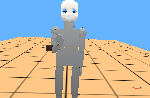effects of the initial joint values

damping = 0.2; initial joint angles = 0; initial elbow angle = 0.3 :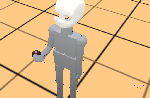damping = 0.1; initial joint angles = 0; initial elbow angle = 0.3 :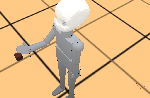cube to far to be reached

damping = 0.1; initial joint angles = 0; initial elbow angle = 0.3 :iCub making a circle

damping = 0.2; initial joint angles = 0; initial elbow angle = 0.3 :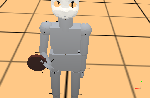damping = 0.1; initial joint angles = 0; initial elbow angle = 0.3 :

No joint limits : damping = 0.1; initial joint angles = 0; initial elbow angle = 0.3 :

## Source code

Contains source code for the kinematic model of the iCub using KDL, and an integration under Webots.
Contains also the new model of the iCub for Webots.